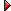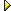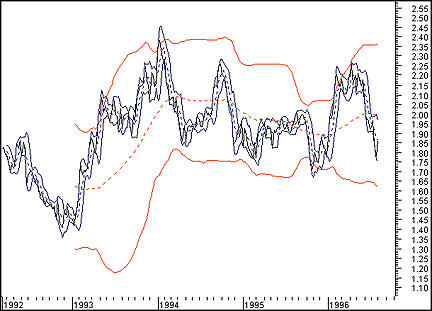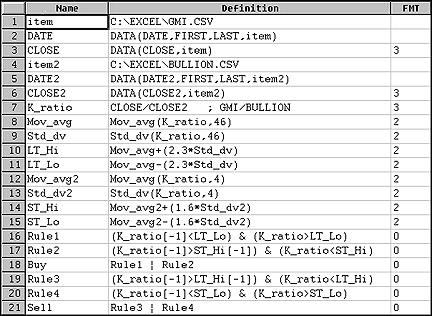Here is this month's selection of Traders' Tips, contributed by various developers of technical analysis software to help readers more easily implement some of the strategies presented in this issue.

You can copy these formulas and programs for easy use in your spreadsheet or analysis software. Simply "select" the desired text by highlighting as you would in any word processing program, then use your standard key command for copy or choose "copy" from the browser menu. The copied text can then be "pasted" into any open spreadsheet or other software by selecting an insertion point and executing a paste command. By toggling back and forth between an application window and the open Web page, data can be transferred with ease.

This month's tips include formulas and programs for:METASTOCK for WindowsTECHNIFILTER PLUSWAVEWI\$E MARKET SPREADSHEETSMARTRADERTRADESTATION

###METASTOCK for Windows

The K-ratio model, which is discussed in Editor Thom Hartle's interview with Nelson Freeburg, is a technique used by Freeburg to generate signals in the precious metals market. The K-ratio model is based on the ratio of Barron's Gold Mining Index (GMI) to gold bullion, over which Bollinger bands are plotted to generate adaptive signals that conform to patterns of price behavior in the precious metals market.

To recreate Freeburg's precious metals switch fund system in MetaStock for Windows, you must first create the K-ratio as a composite security. To do this, launch The DownLoader from Meta-Stock and choose New and then Composite from The DownLoader's File menu. Make sure the directory specified is the directory where your weekly GMI and Handy & Harman data are located. Name the composite "K-ratio," then choose the Barron's GMI as the primary symbol and Handy & Harman prices as the secondary symbol. Next, choose Divide as the operator and then click the OK button to add the composite.Figure 1: METASTOCK. Here's a sample chart of the Bollinger bands used in the precious metals switch system.

Open the K-ratio chart in MetaStock, plot the Bollinger bands indicator and enter 46 for the number of periods and 2.3 for the standard deviations. Plot Bollinger bands again and enter 4 for the periods and 1.6 for the standard deviations. Your chart should look similar to the one in Figure 1.

You can also create this system in MetaStock for DOS using the same steps.

--Allan J. McNichol, Equis International
800 882-3040, 801 265-8886
Internet: http://www.equis.com
GO BACK

###TECHNIFILTER PLUS

Here's a TechniFilter Plus trading strategy that implements Nelson Freeburg's precious metals fund switch system. Formula 1 is the K-ratio calculation, the price of Barron's Gold Mining Index (GMI) divided by the Handy & Harman gold price. Formula 2 computes the 46-week top band, and formula 3 computes the four-week top band. These bands are formed by offsetting a moving average of the K-ratio, which is a multiple of K-ratio standard deviations. Formulas 4 and 5 compute similar bottom bands. Formula 6 is negative if there is a downward crossing of either the top long band or an upward crossing of the lower short band. Formula 7 is positive if there is an upward crossing of the long bottom band or a downward crossing of the short upper band. The signals are based on formulas 6 and 7.
```

Strategy Name: FREEBURG
FORMULAS
1. name: K-RATIO           format: ##.###
formula: ~<GMI>~/~<GOLD>~
2. name: TOP_LONG          format: ##.###
formula: A46 + 2.3*|46
3. name: TOP_SHORT         format: ##.###
formula: A4 + 1.6*|4
4. name: BOT_LONG          format: ##.###
formula: A46 - 2.3*|46
5. name: BOT_SHORT         format: ##.###
formula: A4 - 1.6*|4
6. name: TOP_CROSS         format: ###
formula: ((-)U2-TY1)U3 - ((-)U2-TY1)U2
7. name: BOT_CROSS         format: ###
formula: ((-)U2-TY1)U2 - ((-)U2-TY1)U3
SIGNALS
2. SELL;F6<0
RULES
1. Buy Long 1 at Today's Close on signal: S1
2. Sell Long 1 at Today's Close on signal: S2```
Registered owners of TechniFilter Plus can receive an E-mail copy of these strategies or other TechniFilter Plus formulas, reports and strategies that have appeared in this column by sending a request to rtrsoft@aol.com and noting the item(s) you want. You must include your TechniFilter Plus serial number with your request.
--Clay Burch, RTR Software
919 510-0608
E-mail: rtrsoft@aol.com
GO BACK

###WAVEWI\$E MARKET SPREADSHEET

Here are the WaveWi\$e spreadsheet formulas for implementing Nelson Freeburg's precious metals switch system, described in the interview with Nelson Freeburg:
```

B: GMI
C: Bullion
D: KRatio     GMI / BULLION      'Compute K-Ratio
E: Avg46      @MAVG(KRATIO,46)   'Compute 46 week deviation
bands
F: HiBand46   AVG46+2.3*@STD(KRATIO,46)
G: LoBand46   AVG46-2.3*@STD(KRATIO,46)
H:
I: Avg4       @INIT(45,0); @MAVG(KRATIO,4)
'Compute 4 week deviation
bands
J: HiBand4    AVG4+1.6*@STD(KRATIO,4)
K: LoBand4    AVG4-1.6*@STD(KRATIO,4)
L:
M:
O: Logic      PROCEDURE(RULES)    'Perform Buy / Sell Logic
P:
Q: Profit     @PROFIT(O,P,LONG)   'Compute profits

Procedure Rules
@SIGNAL(B,BUY,KRATIO[-1] < LOBAND46[-1] & KRATIO > LOBAND46 |
KRATIO[-1] > HIBAND4[-1] & KRATIO < HIBAND4,
KRATIO[-1] > HIBAND46[-1] & KRATIO < HIBAND46 |
KRATIO[-1] < LOBAND4[-1] & KRATIO > LOBAND4)```
--Peter Di Girolamo, Jerome Technology
908 369-7503
E-mail: jtiware@aol.com
GO BACK

###SMARTRADER

To implement Nelson Freeburg's dual band trading system used in his K-ratio model, we first obtained a spreadsheet file from STOCKS & COMMODITIES containing pertinent data and extracted subsets of data from it. The Gold Mining Index (GMI) and bullion data were extracted separately and saved as text files consisting of weekly data. Row 7 is the calculation of the K-ratio, GMI divided by gold. A 46-period (week) simple moving average is calculated, as is a 46-period standard deviation. The long-term bands are created as user calculations of +/-2.3 standard deviations away from the moving average. The short-term bands are generated in the same manner.Since this is a dual band system, there are two rules for buy and sell signals. Rule 1 determines that there has been an upward crossing of the K-ratio over the lower long-term band. Rule 2 determines a reverse crossing of the short-term upper band. Buy is a simple "or," which yields a signal if either rule 1 or rule 2 is true. Rule 3 and rule 4 determine a downward crossing of K-ratio through the long upper band or an upward crossing of the lower short-term band. Sell is an "or" using rule 3 and rule 4. Figure 2 shows the rules for the dual band trading system in SmarTrader.

--Jim Ritter, Stratagem Software International
504 885-7353
E-mail: Stratagem1@aol.com
GO BACK

###TRADESTATION

To create the standard error bands described by Jon Andersen in "Standard error bands" in the September 1996 STOCKS & COMMODITIES, you must calculate the beta and alpha coefficients of the linear regression. First, create the calcB and calcA user functions, which refer to the beta and alpha coefficients. Be sure to create and verify these functions before building the indicator. Create calcB first, then calcA. These functions are used in calculating the regression coefficients for the standard error bands.
```

Type: User Function
Name: calcB

Inputs: Length(Numeric);
Vars: X(0);

X = BarNumber;

Value1 = Summation(X * Close, Length) - (Length * Average(X, Length) *Average(Close, Length));
Value2 = Summation(Square(X), Length) - (Length * Square(Average(X, Length)));

calcB = Value1/Value2;

Type: User Function
Name: calcA

Inputs: Length(Numeric);
Vars: X(0);

X = BarNumber;

calcA = Average(Close, Length) - (calcB(Length) * Average(X, Length));```

Next, build the standard error bands indicator. The "length" value specifies the period of the linear regression line; the default is 21. The SDeg value specifies the smoothing factor that is used to smooth the linear regression and standard error bands; the default is 3.

```

Type: Indicator
Name: Std Error Bands Smooth

Inputs: Length(21), SDeg(3);
Vars: LinRegY(0), X(0), StdErr(0), LinRegS(0), SErr(0);

LinRegY = LinearRegValue(Close, Length, 0);
X = CurrentBar;

IF CurrentBar > Length then Begin
Value1 = (Summation(Square(Close), Length)) - ((calcA(Length) *
Summation(Close, Length))) - ((calcB(Length) *
Summation(X * Close, Length)));
Value2 = Length - 2;
StdErr = SquareRoot(Value1 / Value2);
LinRegS = Average(LinRegY, SDeg);
SErr = 2 * Average(StdErr, SDeg);

Plot1(LinRegS, "LinRegS");
Plot2(LinRegS + SErr, "+StdErrS");
Plot3(LinRegS - SErr, "-StdErrS");
End;```

The indicator for the related %A standard error bands is built using the code below. It's best plotted with the bar type set to "histogram."

```

Type: Indicator
Name: Standard Error Bands %A

Inputs: Length(21), SDeg(3);
Vars: LinRegY(0), X(0), StdErr(0), LinRegS(0), SErr(0), PcntA(0);

LinRegY = LinearRegValue(Close, Length, 0);
X = CurrentBar;

IF CurrentBar > Length then Begin
Value1 = (Summation(Square(Close), Length)) - ((calcA(Length) *
Summation(Close, Length))) - ((calcB(Length) *
Summation(X * Close, Length)));
Value2 = Length - 2;
StdErr = SquareRoot(Value1 / Value2);
LinRegS = Average(LinRegY, SDeg);
SErr = 2 * Average(StdErr, SDeg);
PcntA = (Close - (LinRegS-SErr)) / ((LinRegS+SErr)-(LinRegS-SErr)) * 100;

Plot1(PcntA, "%A");
End;```

This code is available at both the Omega Research Forum on America On-Line as well as at Omega's Web site.

-- Gaston Sanchez, Omega Research
Internet: http://www.omegaresearch.com

GO BACK